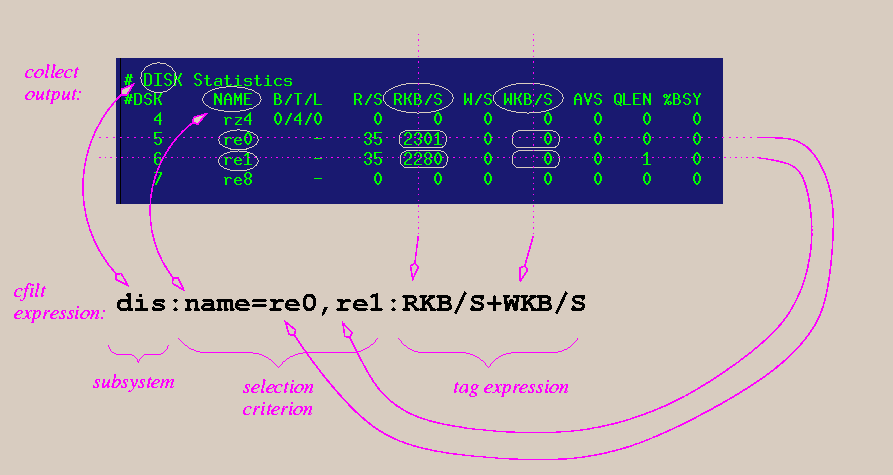### A cfilt example

The following image comprises two main elements: at the top is a block of disk subsystem output from collect. Below it is a cfilt expression.I'll explain the cfilt expression one part at a time.

dis in the ascii output of collect there are 2 or 3 comment lines (starting with '#') before the data for each subsystem. The first line contains the subsystem label. cfilt matches the first three characters of this label with the subsystem specifier in each expression, in this case "dis". this is a selection-criterion. To the left of the equals-sign is some column-tag. To the right is a comma-separated list of values (strings) expected in the given column. Only the lines whose values in the given column match values in the list will be used, and the resulting cfilt output will be in the same order as this list. All other lines will be ignored. Thus the lines with the values "re0" and "re1" will be selected, and the first value will be for "re0", the second for "re1". The column-tag is not case-sensitive, however the values (to the right of the equals-sign) are. That is, they must match exactly. If no selection-criterion is given, all lines are selected, and and summing is automatically turned on. this is the tag-expression. It gets a bit complicated here. The tag-expression is an arbitrary expression consisting of constants, functions (see the manual page for a list), and column-labels, such as "RKB/S". When the expression is evaluated, the actual value in the column under the corresponding column-label is substituted for the column label. So, in this example, for "re0", "RKB/S" is 2301 and "WKB/S" is 0, so the expression is 2301+0, or 2301. The tag-expressions are NOT case-sensitive.

Now I'd like to tie it all together:
For the DISK ("dis") subsystem, all lines are selected which have the values "re0" or "re1" in the "NAME" column. For each selected line the expression "RKB/S+WKB/S" is evaluated. The result of evaluating an expression is written in the corresponding order to cfilt's output. If summing were turned on (by adding a "+" to the end of the subsystem-label (as in "dis+:name=re0,re1:RKB/S+WKB/S"), then for all selected lines (or all lines, if no selection-criterion was given), the results of evaluating the tag-expressions would be summed, producing one value for each expression in the subsystem.

In this example, cfilt's output for this sample would be:
``` <epoch-seconds> <sample-number> 2301 2280 ```